Next: Group Velocity Up: Wave Propagation in Uniform Previous: Sommerfeld Precursor

# Method of Stationary Phase

Equation (887) can be written in the form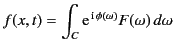(909)

where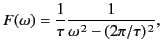(910)

and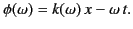(911)

Now,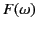is a relatively slowly varying function of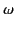(except in the immediate vicinity of the singular points,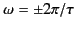), whereas the phase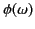is generally large and rapidly varying. The rapid oscillations of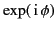over most of the range of integration means that the integrand averages to almost zero. Exceptions to this cancellation rule occur only at points whereis stationary: that is, wherehas an extremum. The integral can therefore be estimated by finding all the points in the-plane wherehas a vanishing derivative, evaluating (approximately) the integral in the neighborhood of each of these points, and summing the contributions. This procedure is known as the method of stationary phase.

Suppose thathas a vanishing first derivative at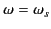. In the neighborhood of this point,can be expanded as a Taylor series,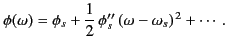(912)

Here, the subscript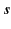is used to indicate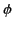, or its second derivative, evaluated at, whereas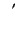denotes a derivative with respect to. Becauseis slowly varying, the contribution to the integral from this stationary phase point is approximately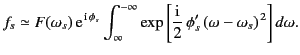(913)

It is tacitly assumed that the stationary point lies on the real axis in-space, so that locally the integral along the contour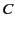is an integral along the real axis in the direction of decreasing. The previous expression can be written in the form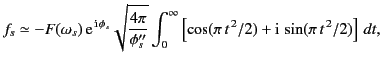(914)

where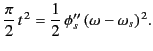(915)

The integrals in the previous expression are known as Fresnel integrals,and can be shown to take the values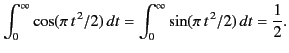(916)

It follows that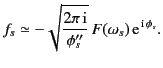(917)

It is easily demonstrated that the arc-length (in the-plane) of the section of the integration contour that makes a significant contribution to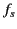is of order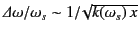. Thus, the arc-length is relatively short, provided that the wavelength of the signal is much less than the distance it has propagated into the dispersive medium. If there is more than one point of stationary phase in the range of integration then the integral is approximated as a sum of terms having the same form as the previous one.

Integrals of the form (910) can be calculated exactly using the method of steepest decent.The stationary phase approximation (918) agrees with the leading term of the method of steepest decent (which is far more difficult to implement than the method of stationary phase) provided thatis real (i.e., provided that the stationary point lies on the real axis). Ifis complex, however, then the stationary phase method can yield erroneous results. This suggests that the stationary phase method is likely to break down when the extremum pointapproaches any poles or branch cuts in the-plane.Next: Group Velocity Up: Wave Propagation in Uniform Previous: Sommerfeld Precursor
Richard Fitzpatrick 2014-06-27